EnchantedLearning.com is a user-supported site.
As a bonus, site members have access to a banner-ad-free version of the site, with print-friendly pages.

 You might also like: V: Illustrated Math Dictionary - Enchanted Learning.com J: Illustrated Math Dictionary - Enchanted Learning.com Q: Illustrated Math Dictionary - Enchanted Learning.com I: Illustrated Math Dictionary - Enchanted Learning.com K: Illustrated Math Dictionary - Enchanted Learning.com Today's featured page: Geology, Rocks and Minerals

 Our subscribers' grade-level estimate for this page: 3rd - 4th

 +, - EnchantedLearning.comMath Dictionary x, ÷
 A B C D E F G H I J K L M N O P Q R S T U V W X Y ZP is for ...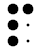MOM2002palindromeA palindrome is a word, phrase or number that reads the same forwards or backwards. A palindromic number is a number that reads the same forwards or backwards, like 2002. Some other palindromes are: the name Bob, the number 101, and the phrase, "Madam, I'm Adam."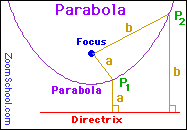parabolaA parabola (a type of conic section) is a curve that is a set of points P such that the distance from a line (the directrix) to P is equal to the distance from P to the focus F. Parabolas have an eccentricity of 1.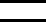parallelParallel lines extend in the same direction, are always the same distance apart, and never intersect (meet).parallelogramA parallelogram is a four-sided figure (a quadrilateral) whose opposite sides are parallel.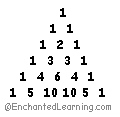Pascal's trianglePascal's triangle is a triangular pattern of numbers devised in 1653 by the French mathematician, physicist, and philosopher Blaise Pascal (June 19, 1623 - Aug. 19, 1662). The numbers in each row are derived by adding pairs of numbers in the row above it. The number one is tacked onto the beginning and end of each row. The first few rows of Pascal's triangle are shown above. The rows represent the binomial coefficients in a triangular format. Note that the row with the numbers [1 2 1] represents the coefficients of (x+y)2. The nth row represents the coefficients of (x+y)n.patternA pattern is something that is repeated, like a design or a series of numbers.pennyA penny is a coin worth one cent. pentadecagonA fifteen-sided polygon is called a pentadecagon.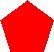pentagonA pentagon is a five-sided polygon. %percentPercent mean "per hundred." The sign for percent is %. 100% means all, 50% means half or 50 out of 100, 25% means one quarter or 25 out of 100, 10% means one tenth or 10 out of 100, and 0% means none or 0 out of 100. To convert a percentage to decimal notation, divide the percentage by 100; for example, 50% = 0.5 and 10% = 0.1. To convert a decimal to a percent, multiply the number by 100; for example, .25 = 25%. perfect numberA perfect number is an integer for which the sum of its proper factors (divisors) is equal to the number itself. For example, 6 is a perfect number because the proper divisors of 6 are 1, 2, and 3 which add up to 6. Compare with abundant number, deficient number.perimeterPerimeter is the distance around the edges of a figure. The perimeter of a square with side length a is P = a+a+a+a = 4 times a. The perimeter of a circle is P = 2πr. The perimeter of a regular polygon is P = ns (where n is the number of sides and s is the side length).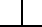perpendicularWhen two lines are perpendicular, they are at right angles (90 degrees). A line can also be perpendicular to a plane (if the line goes through the plane at a 90 degree angle).piPi is the ratio of the circumference of a circle to the diameter of a circle. For any circle, you can divide the circle's circumference (the distance around the circle) by the diameter (the distance across the circle through the center) and you will always get exactly the same number (pi), no matter how big or how small the circle is. Pi is a number roughly equal to 3.14159265... - the digits of pi never end or repeat. The value of pi has been calculated up to the millions of digits. Pi is a transcendental number.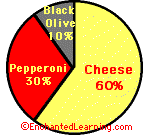pie chartA pie chart (also called a circle graph) is a diagram that is useful for displaying information about the percentages or parts of a whole. Pie charts were invented in 1801 by William Playfair (Sept 22, 1759 - Feb 11, 1823).place valuePlace value is a positional system of notation in which the position of a number with respect to a point determines its value. In the decimal (base ten) system, the value of the digits is based on the number ten.
 planeA plane is a flat (2-dimensional) surface that extends to infinity in all directions. A plane has no thickness.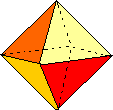Platonic solidShapes that are also called regular polyhedra. The five regular polyhedra that were discovered by the ancient Greeks (and are named for the ancient Greek philosopher Plato). The Pythagoreans knew of the tetrahedron, the cube, and the dodecahedron; the mathematician Theaetetus added the octahedron and the icosahedron. Plato speculated that these five solids were the shapes of the fundamental components of the physical universe.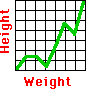plotA plot is a graph done in a coordinate system. Each point on the graph is plotted (marked on the graph in the coordinate system).
 +plus signA plus sign (+) means addition or indicates that a number is positive. .pointA point is an exact location, a spot with no width or thickness.polygonA polygon is a many-sided closed figure with straight edges. A regular polygon is a polygon whose sides are all the same length and whose interior angles are all the same (like an equilateral triangle or a square).polyhedronPolyhedra (the plural of polyhedron) are solids whose faces are polygons. 5x2 - 2x - 8polynomialA polynomial is a sum or difference of terms; each term is: a constant (for example, 5) a constant times a variable (for example, 3x) a constant times the variable to a positive integer power (for example, 2x2) a constant times the product of variables to positive integer powers (for example, 2x3y). A monomial is a polynomial with only one term. A binomial is a polynomial that has two terms. A trinomial is a polynomial with three terms. Degree of a Polynomial: The degree of a term within a polynomial is the sum of the exponents of variables that occur in that term (if there is no exponent written on a variable, such as in 3x, the exponent is one). The degree of a polynomial is the greatest degree of any term in the polynomial (for instance, for the polynomial 4x2 + 7xyz, the degree is 3 because of the last term). If a polynomial isn't just a constant and if each term has at most a variable to the first power (like 4x - 2 or 3y), then it is a first-degree polynomial (also called a linear polynomial). If a polynomial's highest degree is two, it is a quadratic (or second-degree) polynomial (example: 4x2 + 3x + 7). If a polynomial has more than one variable, then its degree is the sum of the exponents of the highest-degree term. For example, the polynomial 2xy2 -3xy + 6x - 2 has degree 3 (the sum of the xy2 exponents, 1 + 2). Polynomials are often written in descending order, in which the terms with the largest powers are written first (like 9x2 - 3x + 6). If they are written with the smallest terms appearing first, this is ascending order (like 6 - 3x + 9x2).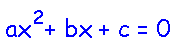polynomial equationA polynomial equation is an equation involving a polynomial.
 positive integerA positive integer is an integer (a whole number) greater than zero, like 1, 2, 3, 4, ... positive numberA positive number is a number greater than zero. Numbers written without a sign are assumed to be positive. For example, 3 = +3. xnpowerA power is the same as an exponent. For example, x to the second power is the same as x squared (x2). A number x to the third power is the same as that number cubed (x3). 2,3,5,7,11,13,17...prime numberA prime number is a positive number that has exactly two factors, 1 and itself. Alternatively, you can think of a prime number as a number greater than one that is not the product of smaller numbers. For example, 13 is a prime number because it can only be divided evenly by 1 and 13. For another example, 14 is not a prime number because it can be divided evenly by 1, 2, 7, and 14. The number one is not a prime number because it has only one factor, 1 itself.
 prismA prism is a solid figure that has two parallel and congruent bases, and its faces are rectangles or parallelograms. probabilityProbability is the chance that a certain event will occur. A probability of 0 (or 0%) means that the event will not occur. A probability of 1 (or 100%) means that the event will definitely occur. For example, the probability that a coin toss will result in heads has a probability of 1/2 (or 50%). The probability that a die throw will result in a six has a probability of 1/6 (or about 17%).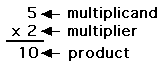productThe product is the answer in a multiplication problem. proofA proof is a logical mathematical argument that demonstrates that a statement is true.protractorA protractor is a device that measures angles.PtolemyClaudius Ptolemaeus or Ptolemy (about 87-150) was a Greek astronomer and mathematician who wrote about his belief that all celestial bodies revolved around the Earth. His writings influenced people's ideas about the universe for over a thousand years, until the Copernican System (with a heliocentric solar system) was accepted.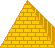pyramidA pyramid is a shape that has a flat polygonal base and triangular sides that meet at a point on the top. The pyramids in Egypt are huge buildings built by ancient Egyptians.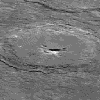PythagorasPythagoras of Samos (569-475 BC) was a Greek philosopher, mathematician, and astronomer who founded a philosophical and religious school, the Pythagorean school in Croton, Italy. Pythagoras believed that the Earth was a sphere at the center of the Universe. He correctly realized that the morning star and the evening star were the same object, the planet Venus. Pythagoras (or the Pythagoreans) made a number of fundamental mathematical discoveries: that for a right triangle, the sum of the squares of the two shorter sides is equal to the square of the hypotenuse (known as the Pythagorean theorem); that the sum of the angles of a triangle is equal to two right angles; and that irrational numbers exist. A 142 km wide lunar crater was named for Pythagoras (Latitude 63.5°, Longitude 63.0°).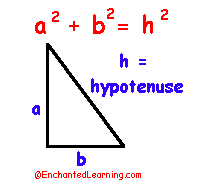Pythagorean theoremThe Pythagorean theorem states that for a right triangle, the sum of the squares of the two shorter sides is equal to the square of the hypotenuse: a2 + b2 = h2.

 +, - EnchantedLearning.comMath Dictionary x, ÷
 A B C D E F G H I J K L M N O P Q R S T U V W X Y Z
 Counting Addition Subtraction Multiplication Division
 Number Line Fractions Decimals Graphs Measurement Rounding

Click on an underlined word for more information on that subject. If the math term you are looking for is not in the dictionary, please e-mail us.

Enchanted Learning®
Over 35,000 Web Pages
Sample Pages for Prospective Subscribers, or click below

 Overview of Site What's New Enchanted Learning Home Monthly Activity Calendar Books to Print Site Index K-3 Crafts K-3 Themes Little ExplorersPicture dictionary PreK/K Activities Rebus Rhymes Stories Writing Cloze Activities Essay Topics Newspaper Writing Activities Parts of Speech Fiction The Test of Time iPhone app TapQuiz Maps - free iPhone Geography Game Biology Animal Printouts Biology Label Printouts Biomes Birds Butterflies Dinosaurs Food Chain Human Anatomy Mammals Plants Rainforests Sharks Whales Physical Sciences: K-12 Astronomy The Earth Geology Hurricanes Landforms Oceans Tsunami Volcano Languages Dutch French German Italian Japanese (Romaji) Portuguese Spanish Swedish Geography/History Explorers Flags Geography Inventors US History Other Topics Art and Artists Calendars College Finder Crafts Graphic Organizers Label Me! Printouts Math Music Word Wheels

Enchanted Learning Search

 Search the Enchanted Learning website for: